# Gravitational Force Formula

Gravitational Force Formula

Every object in the universe attracts every other object. The gravitational force formula, also known as Newton's Law of Gravitation, defines the magnitude of the force between any two objects. The formula for the gravitational force includes the gravitational constant, which has a value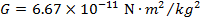. The unit of the gravitational force is Newtons (N).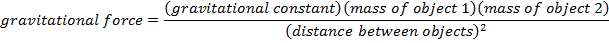Fg = gravitational force between two objects ()

G = gravitational constant ()

m1 = mass of the first object (kg)

m2 = mass of the second object (kg)

r = distance between objects (m)

Gravitational Force Formula Questions:

1) Two satellites orbiting Earth pass close to each other. For a moment, they are 100 m apart. The masses of the satellites are 300 kg and 20.0 kg. What is the magnitude of the force of gravity between these satellites?

Answer: The magnitude of the force of gravity between the satellites can be found using the gravitational force formula: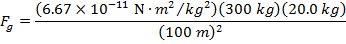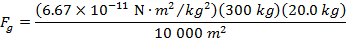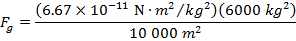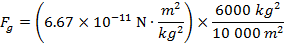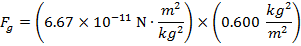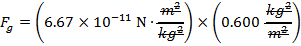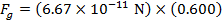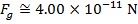The magnitude of the gravitational force between the two satellites when they are 100 m apart is 4.00 x 10-11 N (Newtons).

2) An experiment to measure the force of gravity is set up, using two large spheres. The spheres are both 1000.0 kg, and their centers of mass are 2.000 m apart. What is the force of gravity between these two spheres?

Answer: The magnitude of the force of gravity between the spheres can be found using the gravitational force formula: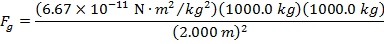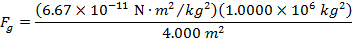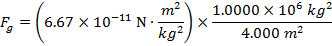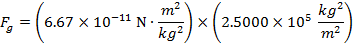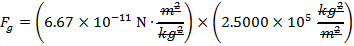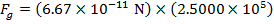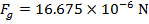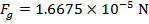The magnitude of the gravitational force between the two spheres is 1.6675 x 10-5 N (Newtons).